# Producer Surplus Definition

Table of Contents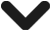# Producer Surplus Definition

## What is Producer Surplus

The producer surplus is the difference between what the producer sells its goods for and the minimum price it would be willing to sell for. In other words, because the producer is selling at a higher price than they would accept, a ‘producer surplus’ is created. The surplus itself is the difference between the two values.

The producer surplus is the other half of the economic surplus, which is also made up of the consumer surplus. Together, they represent the total economic benefit to society.

##### Key Points
1. Producer surplus is the amount that the producer benefits from selling above the price they would otherwise be willing to sell for.
2. The producer surplus can be calculated by taking total revenue and subtracting total cost.
3. Producer surplus and consumer surplus both amount to the total benefit to society – otherwise known as the economic surplus.

If we take an example of producer surplus – McDonald’s may be willing to sell a Big Mac for \$4. Perhaps this is how much it costs the company to make. Yet customers are willing to pay \$7 for it. Therefore, McDonald’s is receiving a producer surplus of \$3 – as this is the difference between what the company is willing to sell for, and what the consumer actually pays.

## How to Calculate Producer Surplus

The producer surplus refers to the area between price and the supply curve. If we look at the graph below, this is the area shaded in grey. This refers to the total producer surplus – so the surplus for all businesses in the market.

To explain, there may be hundreds of thousands of businesses in the market. Some may have a producer surplus of \$3, which is the difference between the minimum value and the price the good is sold at. Other businesses may only have a producer surplus of \$1 – as it costs them \$4 to produce. This would mean they are further up of the supply curve. Each individual business will have a minimum they are willing and able to sell for. Usually, this is the marginal cost. Anything above the marginal cost can be seen as producer surplus – because the firm is selling above the price it costs to produce.

The producer surplus can therefore be calculated using the formula:

Producer Surplus = Final Price – Marginal Cost

## Producer Surplus Graph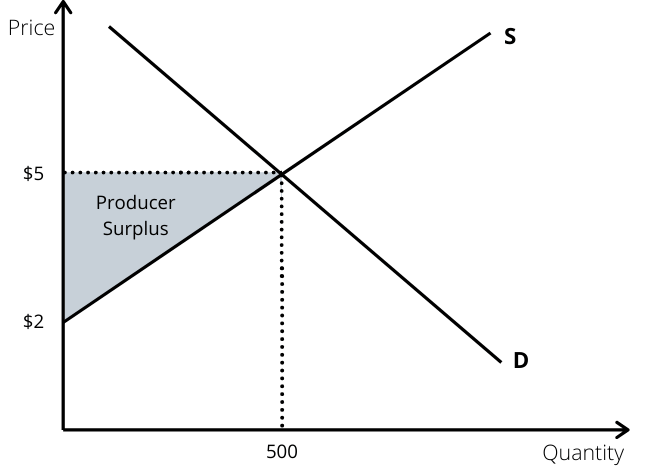## Producer Surplus Formula

To calculate the total producer surplus, we must find the area of the triangle which is below the price, but above the supply curve. In order to calculate this, we use the following formula: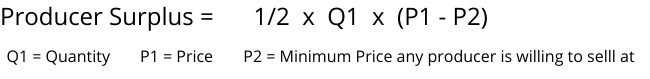This simply calculates the square area between quantity and price, and then half this to find the area of the triangle. This formula calculates to total producer surplus – so the surplus for all the companies operating in a market.

If we want to find out the producer surplus on an individual business level, we can do this using the formula: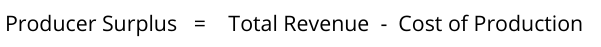Quite simply, this is the profit that the individual business makes. After all, if the business is not making a profit, it will need to stop production. So anything it makes a profit on is essentially a producer surplus.

## Producer Surplus Example

In Country A, there are 500 coffee producers. Each firm produces coffee at a slightly different cost. For some, it costs \$2 to produce, whilst it costs others \$3 and a few pays \$5.

At the equilibrium point, the coffee is sold at \$5 – where supply and demand meet. The producer surplus refers to all those who produce at a cost lower than \$5. The companies that produce at a cost of \$5 make a loss instead of a surplus.

In this example, some companies that are producing at a cost of \$2 are making a surplus of \$3. Others that cost \$4 to produce, are making a surplus of \$1 – the difference between the cost of production and the price.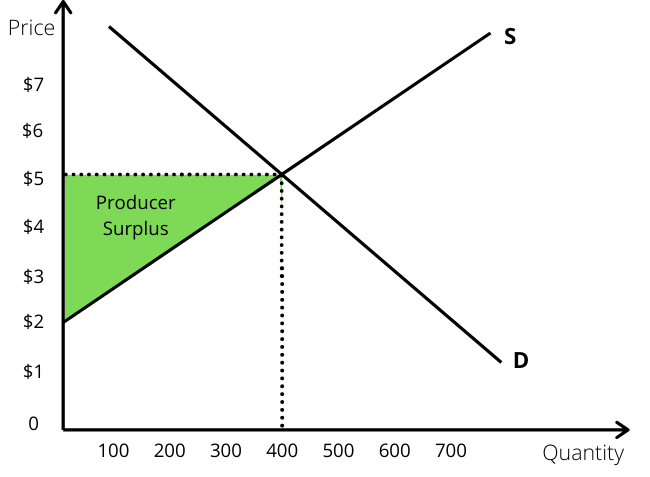On an individual business level, the producer surplus can vary, but the total surplus is shown on the graph in green. In this example, 400 bags of coffee are sold when the price is \$5. That means hundreds of coffee producers obtain a surplus as it reflects the whole market.

To calculate to total surplus, we find out the quantity. In this case, 400 are sold. Then, we calculate the maximum surplus that is achieved. In this case, it is the price sold at, \$5, take away the minimum price a producer would sell, \$2 – which equals \$3. The formula then equals 400 x \$3, which equals \$1,200. We then need to half this as it is a triangle, which brings the final producer surplus to \$600.

## FAQs on Producer Surplus

What is producer surplus?

The producer surplus is the difference between what the producer sells its goods for and the minimum price it would be willing to sell for.

Is producer surplus good or bad?

A producer surplus is good in the fact that it creates a profit for the producer. In turn, this creates an incentive for them to continue production. Without such, firms would not serve the market with many going out of business.

What is producer surplus formula?

On an individual business level, producer surplus can be calculated using the formula: Producer surplus = total revenue – total cost.
On a macro level, we need to calculate the area beneath the price and above the supply curve. This is similar as to how you would calculate the area of a triangle:3 Types and 7 Causes of Monopoly’s - When looking at the causes of monopoly, it is important to first define what it is. The term monopoly originates…Relative Poverty Definition - Relative Poverty occurs when households earn less than 60 percent of the average wage. This means that they have an…Marginal Propensity to Consume Definition - Marginal propensity to consume refers to the percentage of the additional income that is spent. In other words, if a…